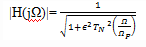Courses

Test: Chebyshev Filters - 2

10 Questions MCQ Test Digital Signal Processing | Test: Chebyshev Filters - 2

Description
Attempt Test: Chebyshev Filters - 2 | 10 questions in 10 minutes | Mock test for Electrical Engineering (EE) preparation | Free important questions MCQ to study Digital Signal Processing for Electrical Engineering (EE) Exam | Download free PDF with solutions
QUESTION: 1

What is the value of |TN(±1)|?

Solution:

Explanation: We know that a chebyshev polynomial of degree N is defined as
TN(x) = cos(Ncos-1x), |x|≤1
cosh(Ncosh-1x), |x|>1
Thus |TN(±1)|=1.

QUESTION: 2

The chebyshev polynomial is oscillatory in the range |x|<∞.

Solution:

Explanation: The chebyshev polynomial is oscillatory in the range |x|≤1 and monotonic outside it.

QUESTION: 3

If NB and NC are the orders of the Butterworth and Chebyshev filters respectively to meet the same frequency specifications, then which of the following relation is true?

Solution:

Explanation: The equi-ripple property of the chebyshev filter yields a narrower transition band compared with that obtained when the magnitude response is monotone. As a consequence of this, the order of a chebyshev filter needed to achieve the given frequency domain specifications is usually lower than that of a Butterworth filter.

QUESTION: 4

The chebyshev-I filter is equi-ripple in pass band and monotonic in the stop band.

Solution:

Explanation: There are two types of chebyshev filters. The Chebyshev-I filter is equi-ripple in the pass band and monotonic in the stop band and the chebyshev-II filter is quite opposite.

QUESTION: 5

What is the equation for magnitude frequency response |H(jΩ)| of a low pass chebyshev-I filter?

Solution:

Explanation: The magnitude frequency response of a low pass chebyshev-I filter is given bywhere ϵ is a parameter of the filter related to the ripple in the pass band and TN(x) is the Nth order chebyshev polynomial.

QUESTION: 6

What is the number of minima’s present in the pass band of magnitude frequency response of a low pass chebyshev-I filter of order 4?

Solution:

Explanation: In the magnitude frequency response of a low pass chebyshev-I filter, the pass band has 2 maxima and 2 minima(order 4=2 maxima+2 minima).

QUESTION: 7

What is the number of maxima present in the pass band of magnitude frequency response of a low pass chebyshev-I filter of order 5?

Solution:

Explanation: In the magnitude frequency response of a low pass chebyshev-I filter, the pass band has 3 maxima and 2 minima(order 5=3 maxima+2 minima).

QUESTION: 8

The sum of number of maxima and minima in the pass band equals the order of the filter.

Solution:

Explanation: In the pass band of the frequency response of the low pass chebyshev-I filter, the sum of number of maxima and minima is equal to the order of the filter.

QUESTION: 9

The poles of HN(s).HN(-s) are found to lie on:

Solution:

Explanation: The poles of HN(s).HN(-s) is given by the characteristic equation 1+ϵ2TN2(s/j)=0.
The roots of the above characteristic equation lies on ellipse, thus the poles of HN(s).HN(-s) are found to lie on ellipse.

QUESTION: 10

If the discrimination factor ‘d’ and the selectivity factor ‘k’ of a chebyshev I filter are 0.077 and 0.769 respectively, then what is the order of the filter?

Solution:

Explanation: We know that the order of a chebyshev-I filter is given by the equation,
N=cosh-1(1/d)/cosh-1(1/k)=4.3
Rounding off to the next large integer, we get N=5.Use Code STAYHOME200 and get INR 200 additional OFF Use Coupon Code

Track your progress, build streaks, highlight & save important lessons and more!

Similar ContentRelated tests